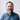# Recreating Elixir's Enum.sum

I’m learning Elixir, and today as an exercise, I was challenged to recreate `Enum.sum` using recursion.

For those new to the language, `Enum.sum` iterates over a list and returns the sum. Here’s an example:

``````iex(0)> Enum.sum([1,2,3,4,5])
15``````

Here is my implementation:

``````defmodule MyList do
@moduledoc """
Defines the list struct and functions.
"""

@doc """
Add all the items in a list together.
"""
def sum(list) do
sum(list, 0)
end

defp sum([], count) do
count
end

defp sum([h|t], count) do
sum(t, count + h)
end
end``````

And the execution:

``````iex(1)> MyList.sum([1,2,3,4,5])
15``````

### Explanation

Elixir doesn’t have loops, so `sum` must be written using recursion. When `MyList.sum([1,2,3,4,5]` is called, here’s what happens.

On the first call, the argument is a non-empty list, so the first (public) `sum` function is invoked. This calls `sum` again, with a count of `0` as an argument.

On the second call, the argument is a non-empty list plus a count, so the last (private) `sum` function is invoked. Here’s where the recursion happens. This function calls itself with the tail (indexes `1` to `-1`) of the list, and adds the head of the list to the count.

This recursion continues until the list is empty, when the second (private) `sum` method is called. This method just returns the count.

A nice side effect of this implementation is that `MyList.sum([])` returns zero, just like `Enum.sum([])`.

http://elixir-lang.org/docs/stable/elixir/Enum.html

Thanks to Josh Branchaud for pointing out some optimizations I have included in this example.